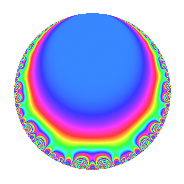# Properties

 Label 24.3.eLevel 24 Weight 3 Character orbit e Rep. character $$\chi_{24}(17,\cdot)$$ Character field $$\Q$$ Dimension 2 Newforms 1 Sturm bound 12 Trace bound 0

# Related objects

## Defining parameters

 Level: $$N$$ = $$24 = 2^{3} \cdot 3$$ Weight: $$k$$ = $$3$$ Character orbit: $$[\chi]$$ = 24.e (of order $$2$$ and degree $$1$$) Character conductor: $$\operatorname{cond}(\chi)$$ = $$3$$ Character field: $$\Q$$ Newforms: $$1$$ Sturm bound: $$12$$ Trace bound: $$0$$

## Dimensions

The following table gives the dimensions of various subspaces of $$M_{3}(24, [\chi])$$.

Total New Old
Modular forms 12 2 10
Cusp forms 4 2 2
Eisenstein series 8 0 8

## Trace form

 $$2q$$ $$\mathstrut +\mathstrut 2q^{3}$$ $$\mathstrut -\mathstrut 12q^{7}$$ $$\mathstrut -\mathstrut 14q^{9}$$ $$\mathstrut +\mathstrut O(q^{10})$$ $$2q$$ $$\mathstrut +\mathstrut 2q^{3}$$ $$\mathstrut -\mathstrut 12q^{7}$$ $$\mathstrut -\mathstrut 14q^{9}$$ $$\mathstrut +\mathstrut 20q^{13}$$ $$\mathstrut +\mathstrut 32q^{15}$$ $$\mathstrut +\mathstrut 4q^{19}$$ $$\mathstrut -\mathstrut 12q^{21}$$ $$\mathstrut -\mathstrut 14q^{25}$$ $$\mathstrut -\mathstrut 46q^{27}$$ $$\mathstrut -\mathstrut 44q^{31}$$ $$\mathstrut +\mathstrut 32q^{33}$$ $$\mathstrut -\mathstrut 12q^{37}$$ $$\mathstrut +\mathstrut 20q^{39}$$ $$\mathstrut +\mathstrut 164q^{43}$$ $$\mathstrut +\mathstrut 64q^{45}$$ $$\mathstrut -\mathstrut 26q^{49}$$ $$\mathstrut -\mathstrut 128q^{51}$$ $$\mathstrut -\mathstrut 64q^{55}$$ $$\mathstrut +\mathstrut 4q^{57}$$ $$\mathstrut -\mathstrut 172q^{61}$$ $$\mathstrut +\mathstrut 84q^{63}$$ $$\mathstrut +\mathstrut 4q^{67}$$ $$\mathstrut -\mathstrut 64q^{69}$$ $$\mathstrut +\mathstrut 164q^{73}$$ $$\mathstrut -\mathstrut 14q^{75}$$ $$\mathstrut +\mathstrut 20q^{79}$$ $$\mathstrut +\mathstrut 34q^{81}$$ $$\mathstrut +\mathstrut 256q^{85}$$ $$\mathstrut +\mathstrut 96q^{87}$$ $$\mathstrut -\mathstrut 120q^{91}$$ $$\mathstrut -\mathstrut 44q^{93}$$ $$\mathstrut -\mathstrut 188q^{97}$$ $$\mathstrut +\mathstrut 64q^{99}$$ $$\mathstrut +\mathstrut O(q^{100})$$

## Decomposition of $$S_{3}^{\mathrm{new}}(24, [\chi])$$ into irreducible Hecke orbits

Label Dim. $$A$$ Field CM Traces $q$-expansion
$$a_2$$ $$a_3$$ $$a_5$$ $$a_7$$
24.3.e.a $$2$$ $$0.654$$ $$\Q(\sqrt{-2})$$ None $$0$$ $$2$$ $$0$$ $$-12$$ $$q+(1+\beta )q^{3}-2\beta q^{5}-6q^{7}+(-7+2\beta )q^{9}+\cdots$$

## Decomposition of $$S_{3}^{\mathrm{old}}(24, [\chi])$$ into lower level spaces

$$S_{3}^{\mathrm{old}}(24, [\chi]) \cong$$ $$S_{3}^{\mathrm{new}}(12, [\chi])$$$$^{\oplus 2}$$